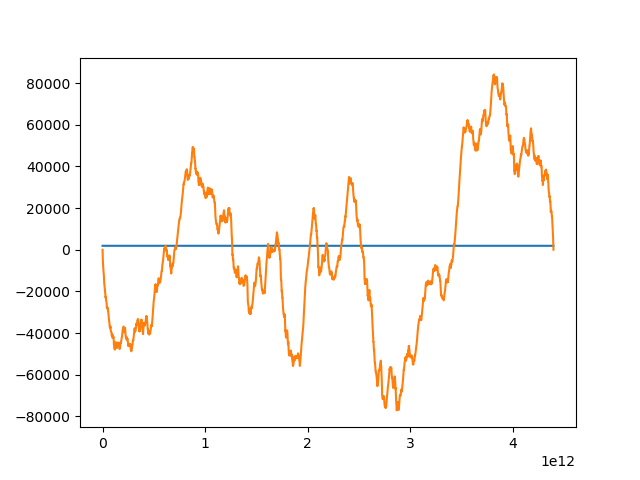# Confused about why model isn't predicting correctly

I’m trying to write a model that will try to approximate the underlying function behind some data (I have a lot of x,y pairs).

Here is the model architecture:

``````class Net(torch.nn.Module):
def __init__(self):
super(Net, self).__init__()
self.fc1 = Linear(1, 50)
self.fc2 = Linear(50, 1)

def forward(self, x):
sigmoid = torch.nn.Sigmoid()
x = self.fc1(x)
x = sigmoid(x)
x = self.fc2(x)
return x

model = Net()
``````

The X values are in the numpy array X = [, , …], the Y values are in a numpy array “res” formatted res = [[0.0], [-652.2099712329232],…].

And here is how I am attempting to training it:

``````# parameters
num_epochs = 200
learning_rate = 0.1

# define the loss function
critereon = L1Loss()

# define the optimizer
optimizer = Rprop(model.parameters(), lr=learning_rate)

predictions = []

# training loop
for epoch in range(num_epochs):
epoch_loss = 0
predictions = []

for ix in range(len(X)):
y_pred = model(Variable(Tensor(X[ix])))
# print(X[ix], res[ix], y_pred.item())
predictions.append(y_pred.item())

loss = critereon(y_pred, Variable(Tensor(res[ix])))
epoch_loss += loss.data.item()

loss.backward()
optimizer.step()

epoch_loss = epoch_loss/len(X)
print("Epoch: {} Loss: {}".format(epoch, epoch_loss))

plt.plot(X,predictions)
plt.plot(X,res)
plt.show()
``````

The final three lines of that snippet are so I can see how the predictions during the final training loop compare to the true Y values. Orange are the true values, blue are the predictions.

The loss values decrease over the course of training, and the predictions do improve after each round of training.

Now, I am trying to predict using this model, doing the following:

``````preds = []
for idx in range(len(X)):
pred = model(Variable(Tensor(X[idx])))
print(X[idx], res[idx], pred.item())
preds.append(pred.item())

plt.plot(X,preds)
plt.plot(X,res)
plt.show()
``````

However, when I do this, the model just predicts one value for the entirety of the X values, resulting in this plot:Does anyone have any idea why this is happening? During training, the predictions seems to be correct, but after training, the model just predicts one value for all the inputs. These are the same inputs it was trained on, and the same inputs for the first plot, so I have no idea what is happening.

Both codes look pretty much equal, so it’s weird your model does not output the same values for the same inputs.
Could you post the complete code so that we could have a look at some code bugs or other issues?

1 Like

Of course. Here is the code:

``````import torch
from torch import Tensor
from torch.nn import Linear, L1Loss, MSELoss, functional as F
from torch.optim import SGD, Adam, RMSprop, Rprop
import numpy as np
import matplotlib.pyplot as plt

# parameters
num_epochs = 10
learning_rate = 0.1

# net architecture
class Net(torch.nn.Module):
def __init__(self):
super(Net, self).__init__()
self.fc1 = Linear(1, 50)
self.fc2 = Linear(50, 1)

def forward(self, x):
sigmoid = torch.nn.Sigmoid()
x = self.fc1(x)
x = sigmoid(x)
x = self.fc2(x)
return x

model = Net()

# define the loss function
critereon = L1Loss()

# define the optimizer
optimizer = Rprop(model.parameters(), lr=learning_rate)

data = np.loadtxt("/Users/arun/Desktop/JHU/sapling/rust/sa_ecoli.key.row.sample", usecols=(0,1),skiprows=0, delimiter=" ")
data = data.T

# turn into a ist of lists
X = np.array([[i]for i in data])
y = np.array([[i]for i in data])

# generate residual

dX = X[len(X)-1] - X
# print(dX)
dy = y[len(y)-1] - y
# print(dy)
m = dy/dX
c = y - X*m

# compute residual values
res = []
for idx in range(len(X)):
lineVal = X[idx] * m + c
trueVal = y[idx]
resVal = lineVal - trueVal
res.append([resVal])

# scale data
res = (res - np.min(res))/np.ptp(res)

# plt.plot(X,y)
# plt.show()
# plt.plot(X, res)
# plt.show()

# store the predictions during training
predictions = []

# create our training loop
for epoch in range(num_epochs):
epoch_loss = 0
predictions = []

for ix in range(len(X)):
y_pred = model(Variable(Tensor(X[ix])))
# print(X[ix], res[ix], y_pred.item())
predictions.append(y_pred.item())

loss = critereon(y_pred, Variable(Tensor(res[ix])))
epoch_loss += loss.data.item()

loss.backward()
optimizer.step()

epoch_loss = epoch_loss/len(X)
print("Epoch: {} Loss: {}".format(epoch, epoch_loss))
# print("Epoch: {}".format(epoch))

plt.plot(X,predictions)
plt.plot(X,res)
plt.show()

model.eval()

preds = []
for idx in range(len(X)):
pred = model(Variable(Tensor(X[idx])))
# print(X[idx], res[idx], pred.item())
preds.append(pred.item())

plt.plot(X,preds)
plt.plot(X,res)
plt.show()
``````

The data is here:
https://github.com/arun96/random/blob/master/sa_ecoli.key.row.sample

Any help would be greatly appreciated - I’m at my wits end when it comes to understanding this.

Thanks for the code!

It looks like the model tends to predict a constant value for the data.
Since you are feeding each sample one by one in the training loop and updating the parameters of the model for each sample, you might force the model to update the predictions for each sample.
If you get rid of the for loop and feed the whole data as a batch during training, the predictions will also be constant.

Generally, your values are really high, so that normalizing them should yield better results.
Since you are dealing with values > 1e8, I would assume that the sigmoid output is basically saturated for each input, which is probably the reason for the constant output.

Let me know, if that helps somehow.

Thank you for the suggestions!

How do you feed the whole data in as a batch during training? I’m not entirely sure how to do that.

And I’ll look into normalizing! Right now I’ve been scaling the y-values, but I will normalize the x ones too.

Thank you!

You would just have to pass the whole batch into the model:

``````y_pred = model(torch.tensor(X).float())
``````

Also note, that `Variables` are deprecated, so you can just use tensors in PyTorch `> 0.4.0`.